Custom SearchMultiplication and Division If a radical is written immediately after another radical, multiplication is intended. Sometimes a dot is placed between the radicals, but not always. Thus, eitherx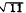ormeans multiplication. When multiplication or division of radicals is indicated, several radicals having the same index can be combined into one radical, if desired. Radicals having the same index are said to be of the SAME ORDER. For example, a is a radical of the second order. The radicalsand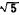are of the same order. If radicals are of the same order, the radicands can be multiplied or divided and placed under one radical symbol. For example,multiplied byis the same as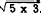. Also,divided byis the same as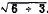. If coefficients appear before the radicals, they also must be included in the multiplication or division. This is illustrated in the following examples:It is important to note that what we have said about multiplication and division does not apply to addition. A typical error is to treat the expression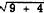as if it were equivalent to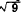+These expressions cannot be equivalent, since 3 + 2 is not equivalent toFACTORING RADICALS.-A radical can be split into two or more radicals of the same order if the radicand can be factored. This is illustrated in the following examples: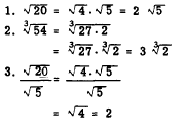SIMPLIFYING RADICALS Some radicals may be changed to an equivalent form that is easier to use. A radical is in its simplest form when no factor can be removed from the radical, when there is no fraction under the radical sign, and when the index of the root cannot be reduced. A factor can be removed from the radical if it occurs a number of times equal to the index of the root. The following examples illustrate this: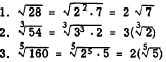Removing a factor that occurs a number of times equal to the index of the root is equivalent to separating a radical into two radicals so that one radicand is a perfect power. The radical sign can be removed from the number that is a perfect square, cube, fourth power, etc. The root taken becomes the coefficient of the remaining radical. In order to simplify radicals easily, it is convenient to know the squares of whole numbers up to about 25 and a few of the smaller powers of the numbers 2, 3, 4, 5, and 6. Table 7-1 shows some frequently used powers of numbers. Table 7-1.-Powers of numbers.Table 7-1.-Powers of numbers-Continued.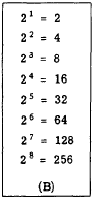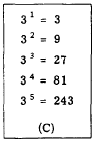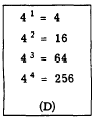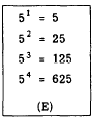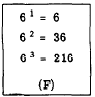Referring to table 7-1 (A), we see that the series of numbers 1, 4, 9, 16, 25, 36, 49, 64, 81, 100 comprises all the perfect squares from 1 to 100 inclusive. If any one of these numbers appears under a square root symbol, the radical sign can be removed immediately. This is illustrated as follows: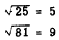A radicand such as 75, which has a perfect square (25) as a factor, can be simplified as follows:This procedure is further illustrated in the following problems: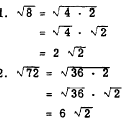By reference to the perfect fourth powers in table 7- 1, we may simplify a radical such as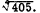. Noting that 405 has the perfect fourth power 81 as a factor, we have the following:As was shown with fractional exponents, taking a root is equivalent to dividing the exponent of a power by the index of the root. If a factor of the radicand has an exponent that is not a multiple of the index of the root, the factor may be separated so that one exponent is divisible by the index, as inConsider alsoIf the radicand is a large number, the perfect powers that are factors are not always obvious. In such a case the radicand can be separated into prime factors. For example,Practice problems. Simplify the radicals and reduce to lowest terms:Answers: